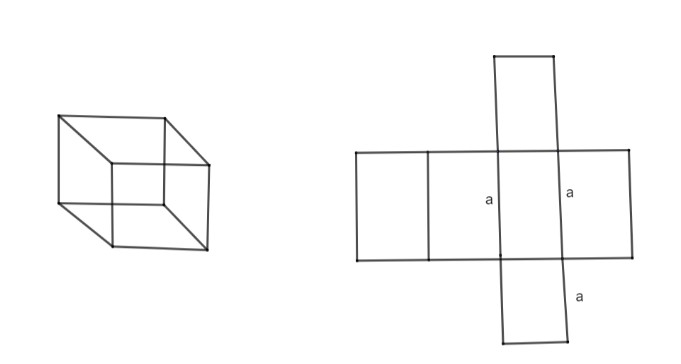Courses
Courses for Kids
Free study material
Free LIVE classes
MoreLIVE
Join Vedantu’s FREE Mastercalss

# The total surface area of a cube is 54$c{{m}^{2}}$. What is the length of its side?Verified
360k+ views
Hint:Cube has 6 faces,the total surface area of the cube is given.Find out the area of one face of the cube and next length of its side.

Given that the total surface area of the cube = 54$c{{m}^{2}}$.
The surface area of a cube is $6{{a}^{2}}$.
Where, a is the length of the side of each edge of the cube.
All the sides of the cube are equal.
$\therefore$a is the length of one side of the cube.By looking at the figure, cube has six sides and each side is a square.
Length of the edge is a
$\therefore$Area of a square$=a\times a={{a}^{2}}$
Since, there are six sides, the total surface area is
Surface area$={{a}^{2}}+{{a}^{2}}+{{a}^{2}}+{{a}^{2}}+{{a}^{2}}+{{a}^{2}}=6{{a}^{2}}$
$\therefore$Surface area of cube is = $6{{a}^{2}}$
We have given the total surface area of cube = 54$c{{m}^{2}}$.
\begin{align} & \Rightarrow 6{{a}^{2}}=54 \\ & \therefore {{a}^{2}}=\dfrac{54}{6} \\ & \Rightarrow {{a}^{2}}=9 \\ & \therefore a=\sqrt{9}=3 \\ \end{align}
Length of its side = 3cm
Note: Cube has 6 faces, so the total surface area becomes $6{{a}^{2}}$.

Last updated date: 18th Sep 2023
Total views: 360k
Views today: 3.60k